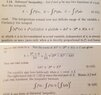• I
• fsonnichsen

#### fsonnichsen

TL;DR Summary
From Margenau and Murphy--quadratic form use not clear
Looking at the proof of the Schwarz inequality in Margenau and Murphy, you will see what I attached. Gamma is asserted to be positive (OK). Given that the usual "quadratic form" solution would read "-(B+B*) .....". The sign does not seem correct to me as shown. In a fact B+B* = 2Re(B) and would be positive in this case given the integrals shown.
What am I missing here?

Thanks
Fritz

#### Attachments

•Margenau .jpg
33.2 KB · Views: 17
•BvU

I think you found an error in the book. However, the values of ##\lambda## don't play a role in the argument (only the sign of the expression under the square root does), so I would call it a small error.

##\ ##

OK and thanks! The authors assumptions make sense but they must be evaluated carefully so I thought I may have missed something 49 years ago when I read the book the 1st time-it was quite a famous book back then.

I find the Cauchy-Schwarz ineq. for complex numbers in N dimensions somewhat tricky-the proof is not intuitively obvious to me upon brief examination.

Take care
Fritz

•WWGD and BvU
Writing
$$\langle f|g \rangle=\int_{\mathbb{R}} \mathrm{d} x f^*(x) g(x),$$
you have from positive definiteness of the scalar product in ##L^2##
$$\langle f+\lambda g|f+\lambda g \rangle \geq 0,$$
and thus for all ##\lambda \in \mathbb{C}##
$$\langle f|f \rangle + \lambda^* \langle g|f \rangle+\lambda \langle f|g \rangle + |\lambda|^2 \langle g|g \rangle \geq 0.$$
Now set ##\lambda=-\langle g|f \rangle/\langle g|g \rangle,##
where we assume that ##g \neq 0## (otherwise the Schwarz inequality holds with the equality sign anyway). With this ##\lambda## the inequality reads
$$\langle f|f \rangle-\frac{|\langle f|g \rangle|^2}{\langle{g} | g\rangle} \geq 0.$$
This obviously is equivalent to
$$|\langle f|g \rangle| \leq \|f \| \|g \|, \quad \text{where} \quad \|f \|=\sqrt{\langle f|f \rangle}.$$
Further, due to the positive definiteness the equality sign holds if and only if there's a ##\lambda## such that ##|f \rangle+\lambda g \rangle=0##, i.e., if ##f \rangle## and ##|g \rangle## are linearly dependent.

•BvU